# Ch08-CryptoConcepts.ppt

28 de May de 2023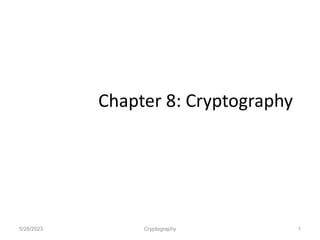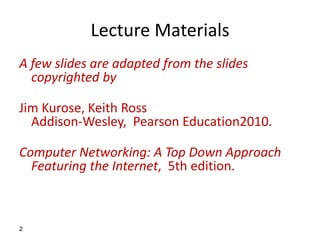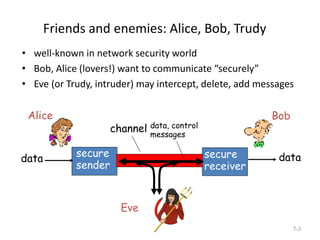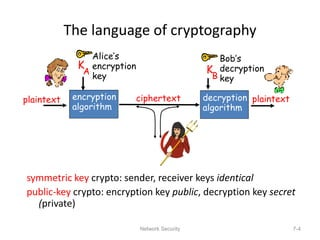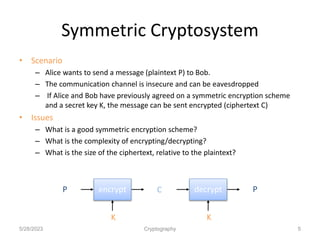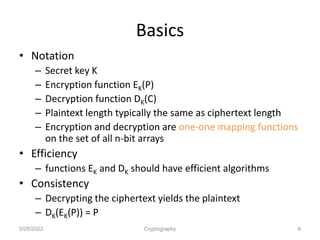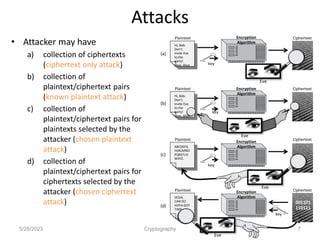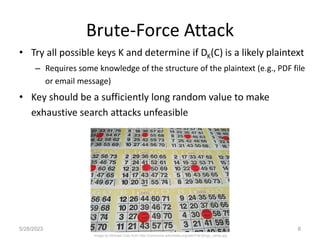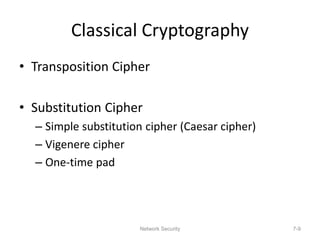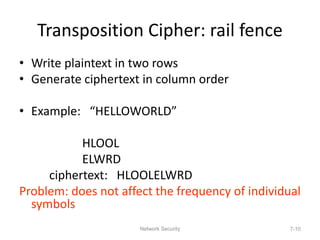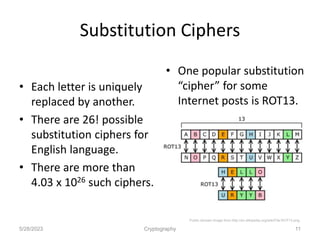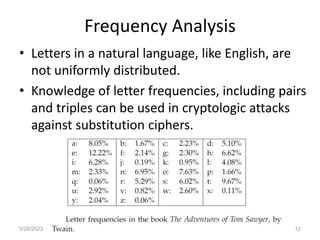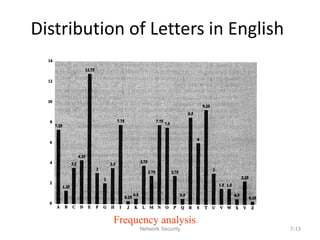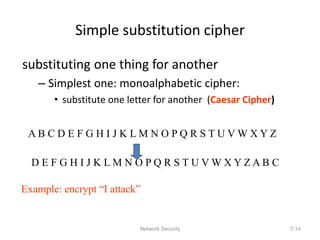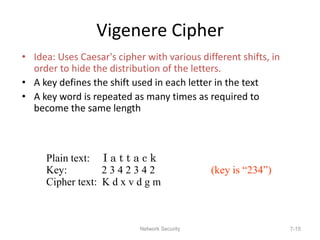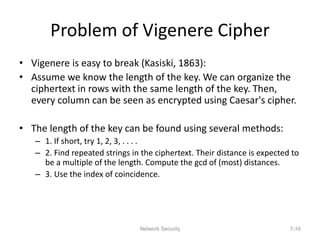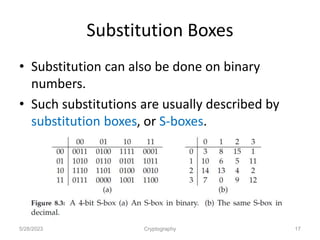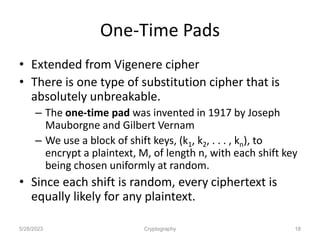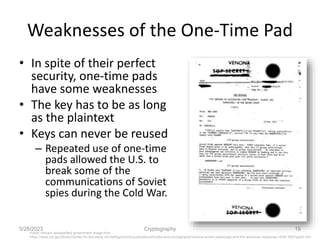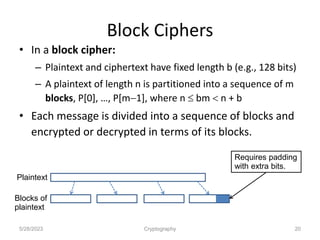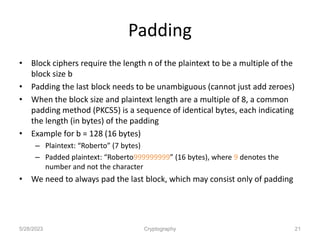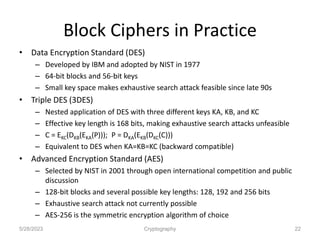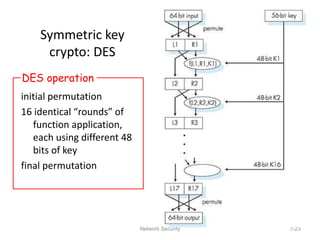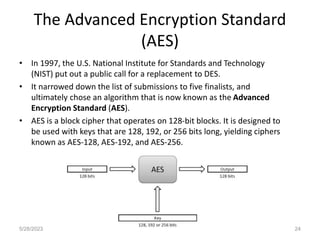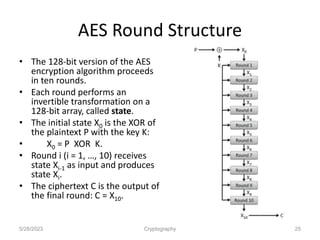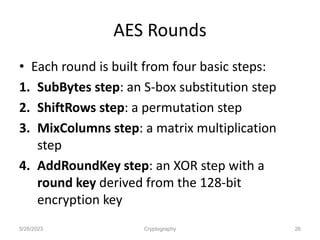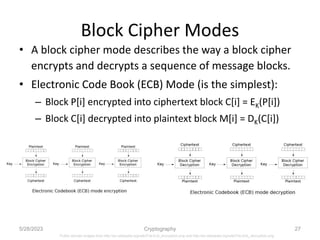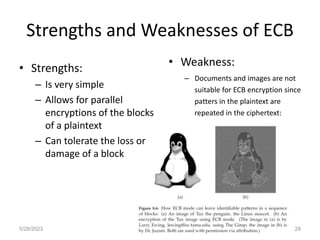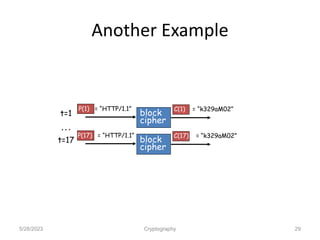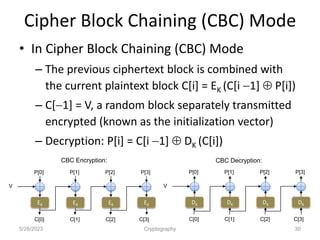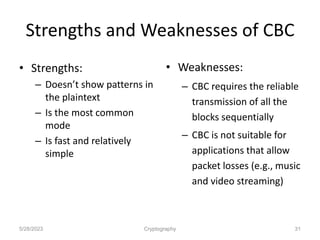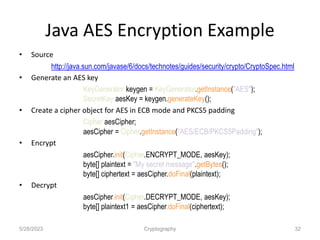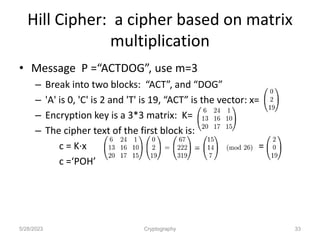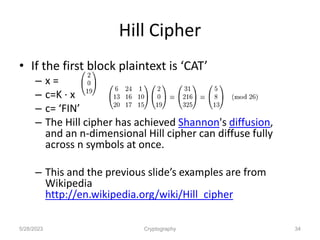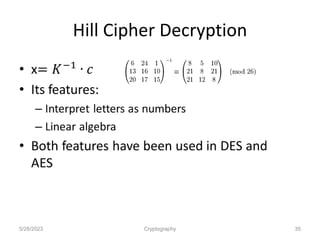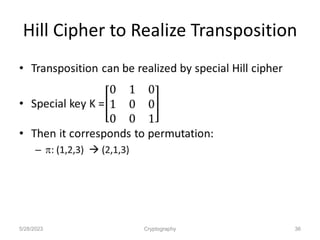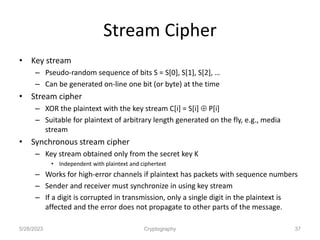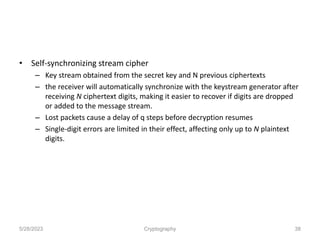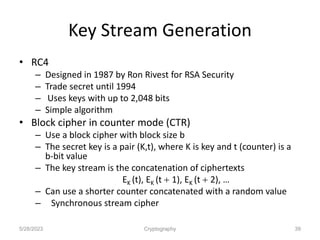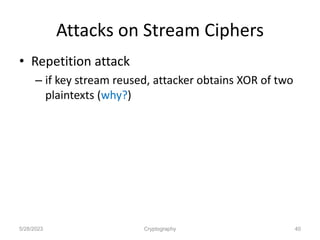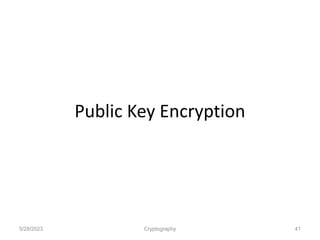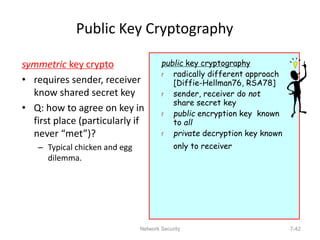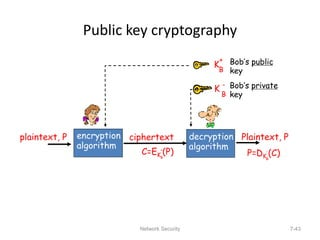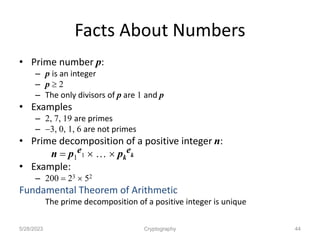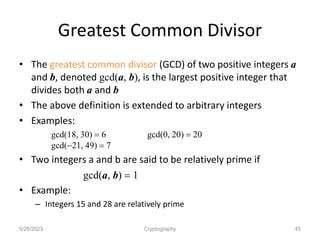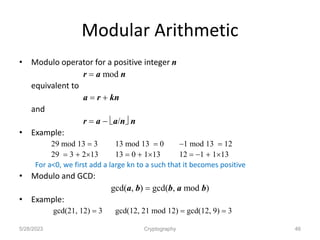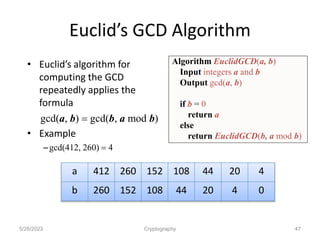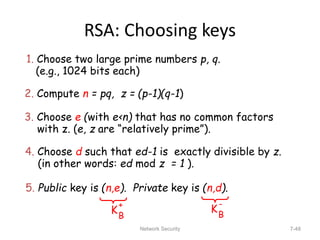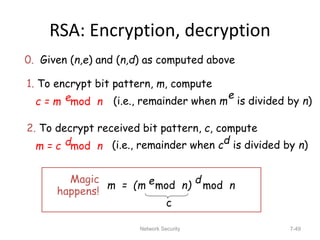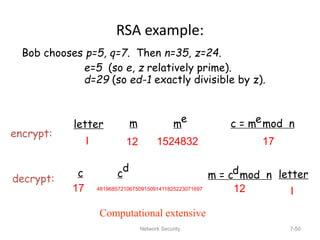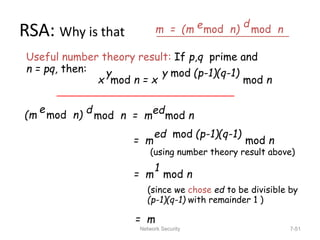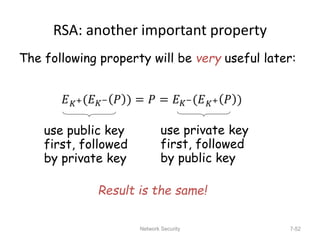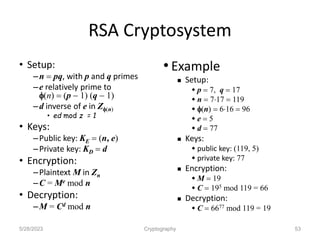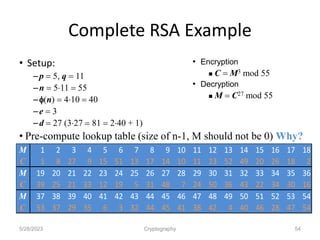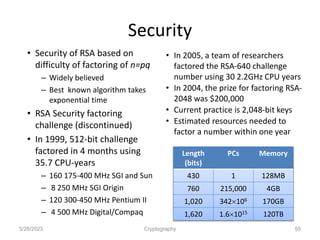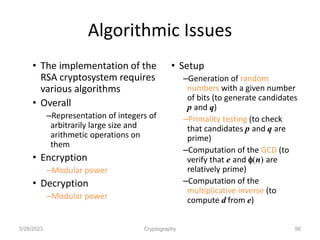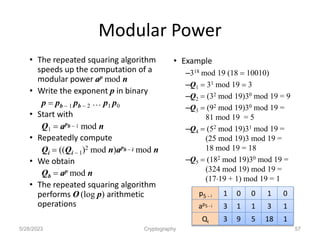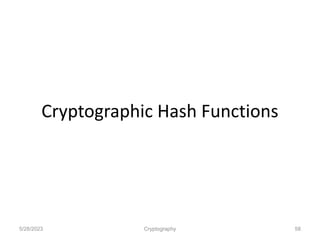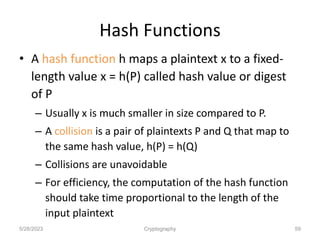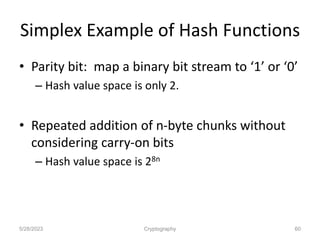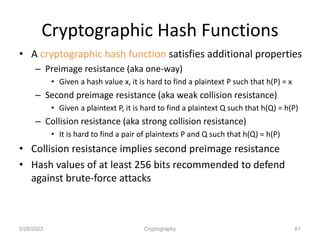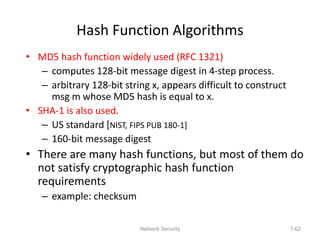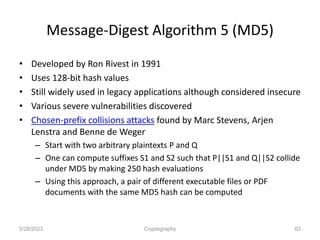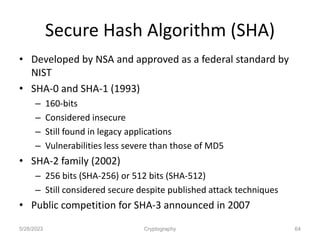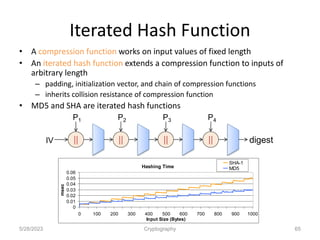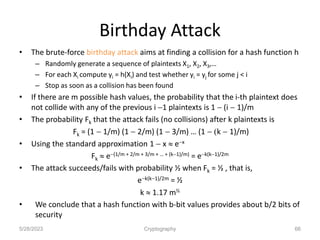1 de 66

### Ch08-CryptoConcepts.ppt

• 2. Lecture Materials A few slides are adapted from the slides copyrighted by Jim Kurose, Keith Ross Addison-Wesley, Pearson Education2010. Computer Networking: A Top Down Approach Featuring the Internet, 5th edition. 2
• 3. 7-3 Friends and enemies: Alice, Bob, Trudy • well-known in network security world • Bob, Alice (lovers!) want to communicate “securely” • Eve (or Trudy, intruder) may intercept, delete, add messages secure sender secure receiver channel data, control messages data data Alice Bob Eve
• 4. Network Security 7-4 The language of cryptography symmetric key crypto: sender, receiver keys identical public-key crypto: encryption key public, decryption key secret (private) plaintext plaintext ciphertext K A encryption algorithm decryption algorithm Alice’s encryption key Bob’s decryption key K B
• 5. Symmetric Cryptosystem • Scenario – Alice wants to send a message (plaintext P) to Bob. – The communication channel is insecure and can be eavesdropped – If Alice and Bob have previously agreed on a symmetric encryption scheme and a secret key K, the message can be sent encrypted (ciphertext C) • Issues – What is a good symmetric encryption scheme? – What is the complexity of encrypting/decrypting? – What is the size of the ciphertext, relative to the plaintext? 5/28/2023 Cryptography 5 C P P encrypt K decrypt K
• 6. Basics • Notation – Secret key K – Encryption function EK(P) – Decryption function DK(C) – Plaintext length typically the same as ciphertext length – Encryption and decryption are one-one mapping functions on the set of all n-bit arrays • Efficiency – functions EK and DK should have efficient algorithms • Consistency – Decrypting the ciphertext yields the plaintext – DK(EK(P)) = P 5/28/2023 Cryptography 6
• 7. Attacks • Attacker may have a) collection of ciphertexts (ciphertext only attack) b) collection of plaintext/ciphertext pairs (known plaintext attack) c) collection of plaintext/ciphertext pairs for plaintexts selected by the attacker (chosen plaintext attack) d) collection of plaintext/ciphertext pairs for ciphertexts selected by the attacker (chosen ciphertext attack) 5/28/2023 Cryptography 7 Hi, Bob. Don’t invite Eve to the party! Love, Alice Encryption Algorithm Plaintext Ciphertext key Eve Hi, Bob. Don’t invite Eve to the party! Love, Alice Plaintext Ciphertext key ABCDEFG HIJKLMNO PQRSTUV WXYZ. Plaintext Ciphertext key IJCGA, CAN DO HIFFA GOT TIME. Plaintext Ciphertext key Eve 001101 110111 (a) (b) (c) (d) Eve Eve Eve Encryption Algorithm Encryption Algorithm Encryption Algorithm
• 8. Brute-Force Attack • Try all possible keys K and determine if DK(C) is a likely plaintext – Requires some knowledge of the structure of the plaintext (e.g., PDF file or email message) • Key should be a sufficiently long random value to make exhaustive search attacks unfeasible 5/28/2023 Cryptography 8 Image by Michael Cote from http://commons.wikimedia.org/wiki/File:Bingo_cards.jpg
• 9. Network Security 7-9 Classical Cryptography • Transposition Cipher • Substitution Cipher – Simple substitution cipher (Caesar cipher) – Vigenere cipher – One-time pad
• 10. Network Security 7-10 Transposition Cipher: rail fence • Write plaintext in two rows • Generate ciphertext in column order • Example: “HELLOWORLD” HLOOL ELWRD ciphertext: HLOOLELWRD Problem: does not affect the frequency of individual symbols
• 11. Substitution Ciphers 5/28/2023 Cryptography 11 • Each letter is uniquely replaced by another. • There are 26! possible substitution ciphers for English language. • There are more than 4.03 x 1026 such ciphers. • One popular substitution “cipher” for some Internet posts is ROT13. Public domain image from http://en.wikipedia.org/wiki/File:ROT13.png
• 12. Frequency Analysis 5/28/2023 Cryptography 12 • Letters in a natural language, like English, are not uniformly distributed. • Knowledge of letter frequencies, including pairs and triples can be used in cryptologic attacks against substitution ciphers.
• 13. Network Security 7-13 Distribution of Letters in English Frequency analysis
• 14. Network Security 7-14 Simple substitution cipher substituting one thing for another – Simplest one: monoalphabetic cipher: • substitute one letter for another (Caesar Cipher) A B C D E F G H I J K L M N O P Q R S T U V W X Y Z D E F G H I J K L M N O P Q R S T U V W X Y Z A B C Example: encrypt “I attack”
• 15. Network Security 7-15 Vigenere Cipher • Idea: Uses Caesar's cipher with various different shifts, in order to hide the distribution of the letters. • A key defines the shift used in each letter in the text • A key word is repeated as many times as required to become the same length Plain text: I a t t a c k Key: 2 3 4 2 3 4 2 (key is “234”) Cipher text: K d x v d g m
• 16. Network Security 7-16 Problem of Vigenere Cipher • Vigenere is easy to break (Kasiski, 1863): • Assume we know the length of the key. We can organize the ciphertext in rows with the same length of the key. Then, every column can be seen as encrypted using Caesar's cipher. • The length of the key can be found using several methods: – 1. If short, try 1, 2, 3, . . . . – 2. Find repeated strings in the ciphertext. Their distance is expected to be a multiple of the length. Compute the gcd of (most) distances. – 3. Use the index of coincidence.
• 17. Substitution Boxes • Substitution can also be done on binary numbers. • Such substitutions are usually described by substitution boxes, or S-boxes. 5/28/2023 Cryptography 17
• 18. One-Time Pads • Extended from Vigenere cipher • There is one type of substitution cipher that is absolutely unbreakable. – The one-time pad was invented in 1917 by Joseph Mauborgne and Gilbert Vernam – We use a block of shift keys, (k1, k2, . . . , kn), to encrypt a plaintext, M, of length n, with each shift key being chosen uniformly at random. • Since each shift is random, every ciphertext is equally likely for any plaintext. 5/28/2023 Cryptography 18
• 19. Weaknesses of the One-Time Pad • In spite of their perfect security, one-time pads have some weaknesses • The key has to be as long as the plaintext • Keys can never be reused – Repeated use of one-time pads allowed the U.S. to break some of the communications of Soviet spies during the Cold War. 5/28/2023 Cryptography 19 Public domain declassified government image from https://www.cia.gov/library/center-for-the-study-of-intelligence/csi-publications/books-and-monographs/venona-soviet-espionage-and-the-american-response-1939-1957/part2.htm
• 20. Block Ciphers • In a block cipher: – Plaintext and ciphertext have fixed length b (e.g., 128 bits) – A plaintext of length n is partitioned into a sequence of m blocks, P, …, P[m1], where n  bm  n + b • Each message is divided into a sequence of blocks and encrypted or decrypted in terms of its blocks. 5/28/2023 Cryptography 20 Plaintext Blocks of plaintext Requires padding with extra bits.
• 21. Padding • Block ciphers require the length n of the plaintext to be a multiple of the block size b • Padding the last block needs to be unambiguous (cannot just add zeroes) • When the block size and plaintext length are a multiple of 8, a common padding method (PKCS5) is a sequence of identical bytes, each indicating the length (in bytes) of the padding • Example for b = 128 (16 bytes) – Plaintext: “Roberto” (7 bytes) – Padded plaintext: “Roberto999999999” (16 bytes), where 9 denotes the number and not the character • We need to always pad the last block, which may consist only of padding 5/28/2023 Cryptography 21
• 22. Block Ciphers in Practice • Data Encryption Standard (DES) – Developed by IBM and adopted by NIST in 1977 – 64-bit blocks and 56-bit keys – Small key space makes exhaustive search attack feasible since late 90s • Triple DES (3DES) – Nested application of DES with three different keys KA, KB, and KC – Effective key length is 168 bits, making exhaustive search attacks unfeasible – C = EKC(DKB(EKA(P))); P = DKA(EKB(DKC(C))) – Equivalent to DES when KA=KB=KC (backward compatible) • Advanced Encryption Standard (AES) – Selected by NIST in 2001 through open international competition and public discussion – 128-bit blocks and several possible key lengths: 128, 192 and 256 bits – Exhaustive search attack not currently possible – AES-256 is the symmetric encryption algorithm of choice 5/28/2023 Cryptography 22
• 23. Network Security 7-23 Symmetric key crypto: DES initial permutation 16 identical “rounds” of function application, each using different 48 bits of key final permutation DES operation
• 24. The Advanced Encryption Standard (AES) • In 1997, the U.S. National Institute for Standards and Technology (NIST) put out a public call for a replacement to DES. • It narrowed down the list of submissions to five finalists, and ultimately chose an algorithm that is now known as the Advanced Encryption Standard (AES). • AES is a block cipher that operates on 128-bit blocks. It is designed to be used with keys that are 128, 192, or 256 bits long, yielding ciphers known as AES-128, AES-192, and AES-256. 5/28/2023 Cryptography 24
• 25. AES Round Structure • The 128-bit version of the AES encryption algorithm proceeds in ten rounds. • Each round performs an invertible transformation on a 128-bit array, called state. • The initial state X0 is the XOR of the plaintext P with the key K: • X0 = P XOR K. • Round i (i = 1, …, 10) receives state Xi-1 as input and produces state Xi. • The ciphertext C is the output of the final round: C = X10. 5/28/2023 Cryptography 25
• 26. AES Rounds • Each round is built from four basic steps: 1. SubBytes step: an S-box substitution step 2. ShiftRows step: a permutation step 3. MixColumns step: a matrix multiplication step 4. AddRoundKey step: an XOR step with a round key derived from the 128-bit encryption key 5/28/2023 Cryptography 26
• 27. Block Cipher Modes • A block cipher mode describes the way a block cipher encrypts and decrypts a sequence of message blocks. • Electronic Code Book (ECB) Mode (is the simplest): – Block P[i] encrypted into ciphertext block C[i] = EK(P[i]) – Block C[i] decrypted into plaintext block M[i] = DK(C[i]) 5/28/2023 Cryptography 27 Public domain images from http://en.wikipedia.org/wiki/File:Ecb_encryption.png and http://en.wikipedia.org/wiki/File:Ecb_decryption.png
• 28. Strengths and Weaknesses of ECB 5/28/2023 Cryptography 28 • Strengths: – Is very simple – Allows for parallel encryptions of the blocks of a plaintext – Can tolerate the loss or damage of a block • Weakness: – Documents and images are not suitable for ECB encryption since patters in the plaintext are repeated in the ciphertext:
• 29. Another Example 5/28/2023 Cryptography 29 t=1 P(1) = “HTTP/1.1” block cipher C(1) = “k329aM02” … t=17 P(17) = “HTTP/1.1” block cipher C(17) = “k329aM02”
• 30. Cipher Block Chaining (CBC) Mode • In Cipher Block Chaining (CBC) Mode – The previous ciphertext block is combined with the current plaintext block C[i] = EK (C[i 1]  P[i]) – C[1] = V, a random block separately transmitted encrypted (known as the initialization vector) – Decryption: P[i] = C[i 1]  DK (C[i]) 5/28/2023 Cryptography 30 DK P DK P DK P DK P V C C C C EK P EK P EK P EK P V C C C C CBC Encryption: CBC Decryption:
• 31. Strengths and Weaknesses of CBC 5/28/2023 Cryptography 31 • Weaknesses: – CBC requires the reliable transmission of all the blocks sequentially – CBC is not suitable for applications that allow packet losses (e.g., music and video streaming) • Strengths: – Doesn’t show patterns in the plaintext – Is the most common mode – Is fast and relatively simple
• 32. Java AES Encryption Example • Source http://java.sun.com/javase/6/docs/technotes/guides/security/crypto/CryptoSpec.html • Generate an AES key KeyGenerator keygen = KeyGenerator.getInstance("AES"); SecretKey aesKey = keygen.generateKey(); • Create a cipher object for AES in ECB mode and PKCS5 padding Cipher aesCipher; aesCipher = Cipher.getInstance("AES/ECB/PKCS5Padding"); • Encrypt aesCipher.init(Cipher.ENCRYPT_MODE, aesKey); byte[] plaintext = "My secret message".getBytes(); byte[] ciphertext = aesCipher.doFinal(plaintext); • Decrypt aesCipher.init(Cipher.DECRYPT_MODE, aesKey); byte[] plaintext1 = aesCipher.doFinal(ciphertext); 5/28/2023 Cryptography 32
• 33. Hill Cipher: a cipher based on matrix multiplication 5/28/2023 Cryptography 33 • Message P =“ACTDOG”, use m=3 – Break into two blocks: “ACT”, and “DOG” – 'A' is 0, 'C' is 2 and 'T' is 19, “ACT” is the vector: x= – Encryption key is a 3*3 matrix: K= – The cipher text of the first block is: c = K∙x = c =‘POH’
• 34. Hill Cipher 5/28/2023 Cryptography 34 • If the first block plaintext is ‘CAT’ – x = – c=K ∙ x – c= ‘FIN’ – The Hill cipher has achieved Shannon's diffusion, and an n-dimensional Hill cipher can diffuse fully across n symbols at once. – This and the previous slide’s examples are from Wikipedia http://en.wikipedia.org/wiki/Hill_cipher
• 36. Hill Cipher to Realize Transposition 5/28/2023 Cryptography 36
• 37. Stream Cipher • Key stream – Pseudo-random sequence of bits S = S, S, S, … – Can be generated on-line one bit (or byte) at the time • Stream cipher – XOR the plaintext with the key stream C[i] = S[i]  P[i] – Suitable for plaintext of arbitrary length generated on the fly, e.g., media stream • Synchronous stream cipher – Key stream obtained only from the secret key K • Independent with plaintext and ciphertext – Works for high-error channels if plaintext has packets with sequence numbers – Sender and receiver must synchronize in using key stream – If a digit is corrupted in transmission, only a single digit in the plaintext is affected and the error does not propagate to other parts of the message. 5/28/2023 Cryptography 37
• 38. • Self-synchronizing stream cipher – Key stream obtained from the secret key and N previous ciphertexts – the receiver will automatically synchronize with the keystream generator after receiving N ciphertext digits, making it easier to recover if digits are dropped or added to the message stream. – Lost packets cause a delay of q steps before decryption resumes – Single-digit errors are limited in their effect, affecting only up to N plaintext digits. 5/28/2023 Cryptography 38
• 39. Key Stream Generation • RC4 – Designed in 1987 by Ron Rivest for RSA Security – Trade secret until 1994 – Uses keys with up to 2,048 bits – Simple algorithm • Block cipher in counter mode (CTR) – Use a block cipher with block size b – The secret key is a pair (K,t), where K is key and t (counter) is a b-bit value – The key stream is the concatenation of ciphertexts EK (t), EK (t + 1), EK (t + 2), … – Can use a shorter counter concatenated with a random value – Synchronous stream cipher 5/28/2023 Cryptography 39
• 40. Attacks on Stream Ciphers • Repetition attack – if key stream reused, attacker obtains XOR of two plaintexts (why?) 5/28/2023 Cryptography 40
• 42. Network Security 7-42 Public Key Cryptography symmetric key crypto • requires sender, receiver know shared secret key • Q: how to agree on key in first place (particularly if never “met”)? – Typical chicken and egg dilemma. public key cryptography r radically different approach [Diffie-Hellman76, RSA78] r sender, receiver do not share secret key r public encryption key known to all r private decryption key known only to receiver
• 43. Network Security 7-43 Public key cryptography plaintext, P ciphertext encryption algorithm decryption algorithm Bob’s public key Plaintext, P C=EK (P) K B + Bob’s private key K B - B + P=DK (C) B -
• 44. Facts About Numbers • Prime number p: – p is an integer – p  2 – The only divisors of p are 1 and p • Examples – 2, 7, 19 are primes – 3, 0, 1, 6 are not primes • Prime decomposition of a positive integer n: n = p1 e1  …  pk ek • Example: – 200 = 23  52 Fundamental Theorem of Arithmetic The prime decomposition of a positive integer is unique 5/28/2023 Cryptography 44
• 45. Greatest Common Divisor • The greatest common divisor (GCD) of two positive integers a and b, denoted gcd(a, b), is the largest positive integer that divides both a and b • The above definition is extended to arbitrary integers • Examples: gcd(18, 30) = 6 gcd(0, 20) = 20 gcd(21, 49) = 7 • Two integers a and b are said to be relatively prime if gcd(a, b) = 1 • Example: – Integers 15 and 28 are relatively prime 5/28/2023 Cryptography 45
• 46. Modular Arithmetic • Modulo operator for a positive integer n r = a mod n equivalent to a = r + kn and r = a  a/n n • Example: 29 mod 13 = 3 13 mod 13 = 0 1 mod 13 = 12 29 = 3 + 213 13 = 0 + 113 12 = 1 + 113 For a<0, we first add a large kn to a such that it becomes positive • Modulo and GCD: gcd(a, b) = gcd(b, a mod b) • Example: gcd(21, 12) = 3 gcd(12, 21 mod 12) = gcd(12, 9) = 3 5/28/2023 Cryptography 46
• 47. Euclid’s GCD Algorithm • Euclid’s algorithm for computing the GCD repeatedly applies the formula gcd(a, b) = gcd(b, a mod b) • Example –gcd(412, 260) = 4 5/28/2023 Cryptography 47 a 412 260 152 108 44 20 4 b 260 152 108 44 20 4 0 Algorithm EuclidGCD(a, b) Input integers a and b Output gcd(a, b) if b = 0 return a else return EuclidGCD(b, a mod b)
• 48. Network Security 7-48 RSA: Choosing keys 1. Choose two large prime numbers p, q. (e.g., 1024 bits each) 2. Compute n = pq, z = (p-1)(q-1) 3. Choose e (with e<n) that has no common factors with z. (e, z are “relatively prime”). 4. Choose d such that ed-1 is exactly divisible by z. (in other words: ed mod z = 1 ). 5. Public key is (n,e). Private key is (n,d). KB + KB -
• 49. Network Security 7-49 RSA: Encryption, decryption 0. Given (n,e) and (n,d) as computed above 1. To encrypt bit pattern, m, compute c = m mod n e (i.e., remainder when m is divided by n) e 2. To decrypt received bit pattern, c, compute m = c mod n d (i.e., remainder when c is divided by n) d m = (m mod n) e mod n d Magic happens! c
• 50. Network Security 7-50 RSA example: Bob chooses p=5, q=7. Then n=35, z=24. e=5 (so e, z relatively prime). d=29 (so ed-1 exactly divisible by z). letter m me c = m mod n e l 12 1524832 17 c m = c mod n d 17 481968572106750915091411825223071697 12 c d letter l encrypt: decrypt: Computational extensive
• 51. Network Security 7-51 RSA: Why is that m = (m mod n) e mod n d (m mod n) e mod n = m mod n d ed Useful number theory result: If p,q prime and n = pq, then: x mod n = x mod n y y mod (p-1)(q-1) = m mod n ed mod (p-1)(q-1) = m mod n 1 = m (using number theory result above) (since we chose ed to be divisible by (p-1)(q-1) with remainder 1 )
• 52. Network Security 7-52 RSA: another important property The following property will be very useful later: use public key first, followed by private key use private key first, followed by public key Result is the same!
• 53. RSA Cryptosystem 5/28/2023 Cryptography 53 • Setup: –n = pq, with p and q primes –e relatively prime to f(n) = (p  1) (q  1) –d inverse of e in Zf(n) • ed mod z = 1 • Keys: –Public key: KE = (n, e) –Private key: KD = d • Encryption: –Plaintext M in Zn –C = Me mod n • Decryption: –M = Cd mod n • Example  Setup:  p = 7, q = 17  n = 717 = 119  f(n) = 616 = 96  e = 5  d = 77  Keys:  public key: (119, 5)  private key: 77  Encryption:  M = 19  C = 195 mod 119 = 66  Decryption:  C = 6677 mod 119 = 19
• 54. Complete RSA Example • Setup: –p = 5, q = 11 –n = 511 = 55 –f(n) = 410 = 40 –e = 3 –d = 27 (327 = 81 = 240 + 1) • Pre-compute lookup table (size of n-1, M should not be 0) Why? 5/28/2023 Cryptography 54 M 1 2 3 4 5 6 7 8 9 10 11 12 13 14 15 16 17 18 C 1 8 27 9 15 51 13 17 14 10 11 23 52 49 20 26 18 2 M 19 20 21 22 23 24 25 26 27 28 29 30 31 32 33 34 35 36 C 39 25 21 33 12 19 5 31 48 7 24 50 36 43 22 34 30 16 M 37 38 39 40 41 42 43 44 45 46 47 48 49 50 51 52 53 54 C 53 37 29 35 6 3 32 44 45 41 38 42 4 40 46 28 47 54 • Encryption  C = M3 mod 55 • Decryption  M = C27 mod 55
• 55. Security • Security of RSA based on difficulty of factoring of n=pq – Widely believed – Best known algorithm takes exponential time • RSA Security factoring challenge (discontinued) • In 1999, 512-bit challenge factored in 4 months using 35.7 CPU-years – 160 175-400 MHz SGI and Sun – 8 250 MHz SGI Origin – 120 300-450 MHz Pentium II – 4 500 MHz Digital/Compaq • In 2005, a team of researchers factored the RSA-640 challenge number using 30 2.2GHz CPU years • In 2004, the prize for factoring RSA- 2048 was \$200,000 • Current practice is 2,048-bit keys • Estimated resources needed to factor a number within one year 5/28/2023 Cryptography 55 Length (bits) PCs Memory 430 1 128MB 760 215,000 4GB 1,020 342106 170GB 1,620 1.61015 120TB
• 56. Algorithmic Issues • The implementation of the RSA cryptosystem requires various algorithms • Overall –Representation of integers of arbitrarily large size and arithmetic operations on them • Encryption –Modular power • Decryption –Modular power • Setup –Generation of random numbers with a given number of bits (to generate candidates p and q) –Primality testing (to check that candidates p and q are prime) –Computation of the GCD (to verify that e and f(n) are relatively prime) –Computation of the multiplicative inverse (to compute d from e) 5/28/2023 Cryptography 56
• 57. Modular Power • The repeated squaring algorithm speeds up the computation of a modular power ap mod n • Write the exponent p in binary p = pb  1 pb  2 … p1 p0 • Start with Q1 = apb  1 mod n • Repeatedly compute Qi = ((Qi  1)2 mod n)apb  i mod n • We obtain Qb = ap mod n • The repeated squaring algorithm performs O (log p) arithmetic operations • Example –318 mod 19 (18 = 10010) –Q1 = 31 mod 19 = 3 –Q2 = (32 mod 19)30 mod 19 = 9 –Q3 = (92 mod 19)30 mod 19 = 81 mod 19 = 5 –Q4 = (52 mod 19)31 mod 19 = (25 mod 19)3 mod 19 = 18 mod 19 = 18 –Q5 = (182 mod 19)30 mod 19 = (324 mod 19) mod 19 = (1719 + 1) mod 19 = 1 5/28/2023 Cryptography 57 p5 - i 1 0 0 1 0 ap5 - i 3 1 1 3 1 Qi 3 9 5 18 1
• 59. Hash Functions • A hash function h maps a plaintext x to a fixed- length value x = h(P) called hash value or digest of P – Usually x is much smaller in size compared to P. – A collision is a pair of plaintexts P and Q that map to the same hash value, h(P) = h(Q) – Collisions are unavoidable – For efficiency, the computation of the hash function should take time proportional to the length of the input plaintext 5/28/2023 Cryptography 59
• 60. Simplex Example of Hash Functions • Parity bit: map a binary bit stream to ‘1’ or ‘0’ – Hash value space is only 2. • Repeated addition of n-byte chunks without considering carry-on bits – Hash value space is 28n 5/28/2023 Cryptography 60
• 61. Cryptographic Hash Functions • A cryptographic hash function satisfies additional properties – Preimage resistance (aka one-way) • Given a hash value x, it is hard to find a plaintext P such that h(P) = x – Second preimage resistance (aka weak collision resistance) • Given a plaintext P, it is hard to find a plaintext Q such that h(Q) = h(P) – Collision resistance (aka strong collision resistance) • It is hard to find a pair of plaintexts P and Q such that h(Q) = h(P) • Collision resistance implies second preimage resistance • Hash values of at least 256 bits recommended to defend against brute-force attacks 5/28/2023 Cryptography 61
• 62. Network Security 7-62 Hash Function Algorithms • MD5 hash function widely used (RFC 1321) – computes 128-bit message digest in 4-step process. – arbitrary 128-bit string x, appears difficult to construct msg m whose MD5 hash is equal to x. • SHA-1 is also used. – US standard [NIST, FIPS PUB 180-1] – 160-bit message digest • There are many hash functions, but most of them do not satisfy cryptographic hash function requirements – example: checksum
• 63. Message-Digest Algorithm 5 (MD5) • Developed by Ron Rivest in 1991 • Uses 128-bit hash values • Still widely used in legacy applications although considered insecure • Various severe vulnerabilities discovered • Chosen-prefix collisions attacks found by Marc Stevens, Arjen Lenstra and Benne de Weger – Start with two arbitrary plaintexts P and Q – One can compute suffixes S1 and S2 such that P||S1 and Q||S2 collide under MD5 by making 250 hash evaluations – Using this approach, a pair of different executable files or PDF documents with the same MD5 hash can be computed 5/28/2023 Cryptography 63
• 64. Secure Hash Algorithm (SHA) • Developed by NSA and approved as a federal standard by NIST • SHA-0 and SHA-1 (1993) – 160-bits – Considered insecure – Still found in legacy applications – Vulnerabilities less severe than those of MD5 • SHA-2 family (2002) – 256 bits (SHA-256) or 512 bits (SHA-512) – Still considered secure despite published attack techniques • Public competition for SHA-3 announced in 2007 5/28/2023 Cryptography 64
• 65. Iterated Hash Function • A compression function works on input values of fixed length • An iterated hash function extends a compression function to inputs of arbitrary length – padding, initialization vector, and chain of compression functions – inherits collision resistance of compression function • MD5 and SHA are iterated hash functions 5/28/2023 Cryptography 65 || || || || P1 P2 P3 P4 IV digest Hashing Time 0 0.01 0.02 0.03 0.04 0.05 0.06 0 100 200 300 400 500 600 700 800 900 1000 Input Size (Bytes) msec SHA-1 MD5
• 66. Birthday Attack • The brute-force birthday attack aims at finding a collision for a hash function h – Randomly generate a sequence of plaintexts X1, X2, X3,… – For each Xi compute yi = h(Xi) and test whether yi = yj for some j < i – Stop as soon as a collision has been found • If there are m possible hash values, the probability that the i-th plaintext does not collide with any of the previous i 1 plaintexts is 1  (i  1)/m • The probability Fk that the attack fails (no collisions) after k plaintexts is Fk = (1  1/m) (1  2/m) (1  3/m) … (1  (k  1)/m) • Using the standard approximation 1  x  ex Fk  e(1/m + 2/m + 3/m + … + (k1)/m) = ek(k1)/2m • The attack succeeds/fails with probability ½ when Fk = ½ , that is, ek(k1)/2m = ½ k  1.17 m½ • We conclude that a hash function with b-bit values provides about b/2 bits of security 5/28/2023 Cryptography 66